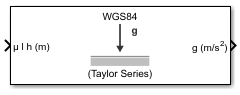# WGS84 Gravity Model

Implement 1984 World Geodetic System (WGS84) representation of Earth's gravity

## Library

Environment/Gravity

•## Description

The WGS84 Gravity Model block implements the mathematical representation of the geocentric equipotential ellipsoid of the World Geodetic System (WGS84). The block output is the Earth's gravity at a specific location. Gravity precision is controlled via the Type of gravity model parameter.

The WGS84 Gravity Model block icon displays the input and output units selected from the Units list.

## Parameters

Type of gravity model

Specifies the method to calculate gravity:

• `WGS84 Taylor Series`

• `WGS84 Close Approximation`

• `WGS84 Exact`

Units

Specifies the input and output units:

UnitsHeightGravity
`Metric (MKS)`MetersMeters per second squared
`English`FeetFeet per second squared
Exclude Earth's atmosphere

Select for the value for the Earth's gravitational field to exclude the mass of the atmosphere.

Clear for the value for the Earth's gravitational field to include the mass of the atmosphere.

This option is available only with Type of gravity model WGS84 Close Approximation or WGS84 Exact.

Precessing reference frame

When selected, the angular velocity of the Earth is calculated using the International Astronomical Union (IAU) value of the Earth's angular velocity and the precession rate in right ascension. To obtain the precession rate in right ascension, Julian centuries from Epoch J2000.0 is calculated using the dialog parameters of Month, Day, and Year.

If cleared, the angular velocity of the Earth used is the value of the standard Earth rotating at a constant angular velocity.

This option is available only with Type of gravity model WGS84 Close Approximation or WGS84 Exact.

Input Julian date

When selected, another input port, JD, appears on the block mask. Select this check box if you want to manually specify the Julian date for the block. Otherwise, the block calculates the Julian date given the values of Month, Day, and Year. The year must be after January 1, 2000 (2451545). Selecting this block disables the Month, Day, and Year parameters.

Month

Specifies the month used to calculate Julian centuries from Epoch J2000.0.

This option is available only with Type of gravity model WGS84 Close Approximation or WGS84 Exact and only when Precessing reference frame is selected. It is disabled if you select Input Julian Date.

Day

Specifies the day used to calculate Julian centuries from Epoch J2000.0.

This option is available only with Type of gravity model WGS84 Close Approximation or WGS84 Exact and only when Precessing reference frame is selected. It is disabled if you select Input Julian Date.

Year

Specifies the year used to calculate Julian centuries from Epoch J2000.0. The year must be 2000 or greater.

This option is available only with Type of gravity model WGS84 Close Approximation or WGS84 Exact and only when Precessing reference frame is selected. It is disabled if you select Input Julian Date.

No centrifugal effects

When selected, calculated gravity is based on pure attraction resulting from the normal gravitational potential.

If cleared, calculated gravity includes the centrifugal force resulting from the Earth's angular velocity.

This option is available only with Type of gravity model WGS84 Close Approximation or WGS84 Exact.

Action for out-of-range input

Specify if out-of-range input invokes a warning, error, or no action.

## Inputs and Outputs

InputDimension TypeDescription

First

Three-element vector or M-by-3 array

Contains the position in geodetic latitude, longitude and altitude, with units in degrees, degrees, and selected units of length respectively. Altitude must be less than 20,000.0 m (approximately 65,620.0 feet).

Second (Optional)

Scalar

Contains the Julian centuries that you specified.

OutputDimension TypeDescription
Output

Three-element vector or M-by-3 array

Gravity in the north-east-down (NED) coordinate system. The Taylor Series and Close Approximation methods output only normal gravity (down in the NED Coordinate system). The Exact method outputs both normal and tangent gravity (down and north in the NED coordinate system).

## Assumptions and Limitations

The WGS84 gravity calculations are based on the assumption of a geocentric equipotential ellipsoid of revolution. Since the gravity potential is assumed to be the same everywhere on the ellipsoid, there must be a specific theoretical gravity potential that can be uniquely determined from the four independent constants defining the ellipsoid.

Use of the WGS84 Taylor Series model should be limited to low geodetic heights. It is sufficient near the surface when submicrogal precision is not necessary. At medium and high geodetic heights, it is less accurate.

The WGS84 Close Approximation model gives results with submicrogal precision.

To predict and determine a satellite orbit with high accuracy, use the EGM96 through degree and order 70.

## Examples

See the Environment Models subsystem in the Airframe subsystem of the `aeroblk_HL20` model for an example of this block.

## Reference

 NIMA TR8350.2: “Department of Defense World Geodetic System 1984, Its Definition and Relationship with Local Geodetic Systems.”

## Extended Capabilities

### C/C++ Code GenerationGenerate C and C++ code using Simulink® Coder™.

#### Introduced before R2006a

##### SupportGet trial now